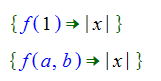# Variables

The variables described here are variables that are used in Sym code. These variables must be in either the Variables or VariableArrays namespaces.

Variables like the "x" and "y" in

x + 3 = y

are variables in the mathematical sense. Typically, they represent an unknown quantity in an equation. The variables considered in this topic are the variables used in Sym code.

Variables used in Sym code are bound to values (which can, in principle, be any expression) by Match objects in Sym clauses (see Pattern Matching - Overview). Mathematical variables can be in almost any namespace, but are most commonly in the Local namespace. Variables in Sym code have to be either in the Variables or in the VariableArrays namespace.

## Simple variables

Simple variables are just objects in the Variables namespace. These variables are bound to an expression by one of the match objects. An example would be:Variables can also have parameters. A variable with parameters will only match an expression with the same number of parameters. Each of the parameters must also match. For example, the following would match:where these matches would fail:See Pattern Matching - Overview for more details.

## List variables

List variables were introduced to allow matching against terms where the number of parameters is different in different instances. For example, the expressions

x + y

and

x + y + z + w

are both instances of the Add object, but the first has two parameters and the second has four parameters. List variables allow you to write pattern matches. See Pattern Matching to Monoids for more details.

## The Question variable

This variable (Variables.Question) is normally rendered as a question mark. It differs from other variables in that values are never bound to the question mark variable. It's equivalent to the underscore used in Haskell and means that you don't care what is at the location in the expression that would have been bound if another variable had been used in place of the Question variable.

One example where the question variable is used is in creating the patterns that go in the second parameter of the FindPattern function.

## The VariableArrays namespace

Since simple variables can have parameters, and the parameters are used in the matching process as described above in the Simple variables section, we don't have a way to construct something equivalent to an array in a normal programming language. To do this, we have introduced a second namespace, the VariableArrays namespace, that also contains variables.

Parameters of variables in this namespace are treated differently than parameters of variables in the Variables namespace. The expressions in the parameters of variables in the VariableArrays namespace are treated as part of the name of the variable. The variable "c(0)" in the the VariableArrays namespace will match against any expression and will bind the variable "c(0)" to that expression. The variable "c(0)" in the Variables namespace will only match expressions that have one parameter where that parameter is the zero object. In that case, the variable "c" will be bound the the parent object without any parameters.